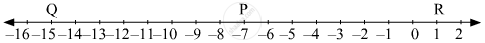Advertisement Remove all ads

# Co-ordinate of Point P on a Number Line is - 7. Find the Co-ordinates of Points on the Number Line Which Are at a Distance of 8 Units from Point P. - Geometry

Sum

Co-ordinate of point P on a number line is - 7. Find the co-ordinates of points on the number line which are at a distance of 8 units from point P.

Advertisement Remove all ads

#### Solution

The co-ordinates of point P on the number line is −7. Now, there will be two points, one on the left of point P and the other on the right of point P on the number line which are at a distance of 8 units from point P.

Let the point R is on the right of point P and point Q is on the left of point P each at a distance of 8 units from point P.

The co-ordinate of point R will be larger and co-ordinate of point Q will be smaller in comparison to the co-ordinate of point P.

Now, d(P, R) = 8
So, co-ordinate of R − co-ordinate of P = 8
∴ co-ordinate of R = 8 + co-ordinate of P = 8 + (−7) = 8 − 7 = 1
Also, d(Q, P) = 8
So, co-ordinate of P − co-ordinate of Q = 8
∴ co-ordinate of Q =  co-ordinate of P − 8 = −7 − 8 = −15Hence, the co-ordinates of the required points on the number line which are at a distance of 8 units from the point P are 1 and −15.

Concept: Co-ordinates of Points and Distance
Is there an error in this question or solution?
Advertisement Remove all ads

#### APPEARS IN

Balbharati Mathematics 2 Geometry 9th Standard Maharashtra State Board
Chapter 1 Basic Concepts in Geometry
Problem set 1 | Q 4 | Page 12
Advertisement Remove all ads
Advertisement Remove all ads
Share
Notifications

View all notifications

Forgot password?